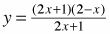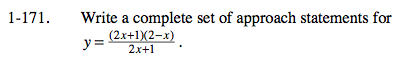### Home > CALC > Chapter 1 > Lesson 1.4.3 > Problem1-171

1-171.

Write a complete set of approach statements for. Homework Help ✎Other than ±∞, what other x value(s) are approached but never reached? Consider when the function's y-value is undefined.

Remember that a value can be approached from both the positive and negative side.

$\text{As }x\rightarrow -\infty ,\ y\rightarrow \infty .$

$\text{As }x\rightarrow \infty ,\ y\rightarrow -\infty .$

$\text{As }x\rightarrow -\frac{1}{2}^{-} ,\ y\rightarrow \frac{5}{2} .$

$\text{As }x\rightarrow -\frac{1}{2}^+ ,\ y\rightarrow \frac{5}{2} .$